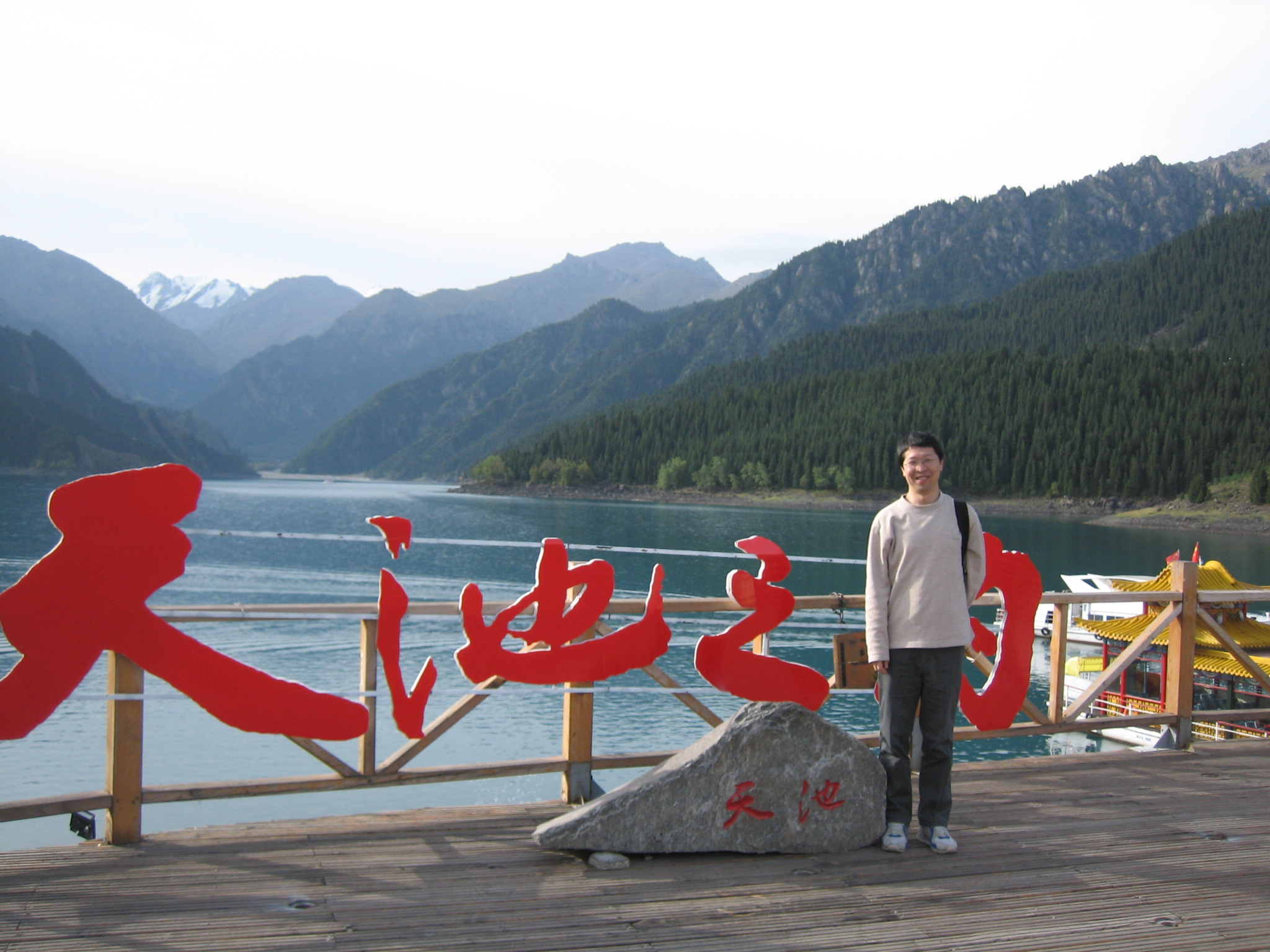Skip to content Skip to navigation

# Yutian Lei ProfessorResearch Interests:
• PDE
• Nonlinear Analysis
Bio:

### Educational Background

• PhD, Pure Mathematic, Jilin University (1999)
• BA, Mathematics, Jilin University, (1993)

### Research

Research Projects

Singular potential theory and qualitative analysis for the static nonlocal Schrodinger equations (2015-2018)

Journal Articles

1. Lei Yutian, Asymptotic estimation for a p-Ginzburg-Landau type minimizer in higher dimensions, Pacific J. Math., Vol.226, (2006), No.1, 103-135.
2. Lei Yutian, Asymptotic estimations for a p-Ginzburg-Landau type minimizer, Math. Nachr., Vol.280, (2007), No.13-14, 1559-1576.
3. Lei Yutian, Singularity analysis of a p-Ginzburg-Landau type minimizer, Bull. Sci. Math., Vol.134 (2010), No.1, 97-115.
4. Lei Yutian, Decay rates for solutions of an integral system of Wolff type, Potential Anal., Vol.35 (2011), No.4, 387-402.
5. Lei Yutian, Li Congming, Ma Chao, Decay estimation for positive solutions of a gamma-Laplace equation, Discrete Contin. Dyn. Syst., Vol.30 (2011), No.2, 547-558.
6. Lei Yutian, Ma Chao, Radial symmetry and decay rates of positive solutions of a Wolff type integral system, Proc. Amer. Math. Soc., Vol.140 (2012), No.2, 541-551.
7. Lei Yutian, Li Congming, Integrability and asymptotics of positive solutions of a gamma-Laplace system, J. Differential Equations, Vol.252 (2012), No.3, 2739-2758.
8. Lei Yutian, Li Congming, Ma Chao, Asymptotic radial symmetry and growth estimates of positive solutions to weighted Hardy-Littlewood-Sobolev system, Calc. Var. Partial Differential Equations, Vol.45 (2012), No.1-2, 43-61.
9. Sun Sha, Lei Yutian, Fast decay estimates for integrable solutions of the Lane-Emden type integral systems involving the Wolff potentials, J. Funct. Anal., Vol.263 (2012), No.12, 3857-3882.
10. Lei Yutian, Lu Zhongxue, Axisymmetry of locally bounded solutions to an Euler-Lagrange system of the weighted Hardy-Littlewood-Sobolev inequality, Discrete Contin. Dyn. Syst., Vol.33 (2013). No.5, 1987-2005.
11. Lei Yutian, On the Regularity of positive solutions of a Choquard type equation, Math. Z., Vol. 273 (2013). No.3-4, 883-905.
12. Lei Yutian, Singularity analysis of Ginzburg-Landau energy related to p-wave superconductivity, Z. Angew. Math. Phys., Vol.64 (2013), No.4, 1249-1266.
13. Lei Yutian, Asymptotic properties of positive solutions of the Hardy-Sobolev type equations, J. Differential Equations, Vol.254 (2013), No.4, 1774-1799.
14. Lei Yutian, Qualitative analysis for the static Hartree type equations, SIAM J. Math. Anal., Vol.45 (2013), No.1, 388-406.
15. Lei Yutian, On the integral systems with negative exponents, Discrete Contin. Dyn. Syst.,Vol.35 (2015), No.3, 1039-1057.
16. Lei Yutian, Wolff type potential estimates and application to nonlinear equations with negative exponents, Discrete Contin. Dyn. Syst., Vol.35 (2015), No.5, 2067-2078.
17. Lei Yutian, Critical conditions and finite energy solutions of several nonlinear elliptic PDEs in Rn, J. Differential Equations, Vol.258 (2015), No.11, 4033-4061.
18. Lei Yutian, Li Congming, Sharp criteria of Liouville type for some nonlinear systems, Discrete Contin. Dyn. Syst., Vol.36 (2016), No.6, 3277-3315.

### Contact

Email：leiyutian@njnu.edu.cn